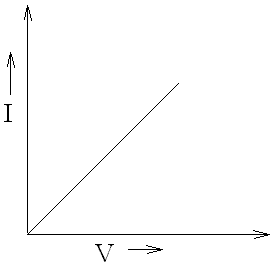Ohm's LawOhm's Law states that, "The current flowing through the conductor is directly proportional to the potential difference across its ends keeping the external physical conditions constant.

i.e.,

V  I          where, V is the potential difference across its ends and I is the current flowing through                                       the conductor.
or V = IR

Here, R is a proportionality constant known as the resistance of the conductor.

Experimental Verification Of Ohm's Law

Here's a simple animated video to get the basic idea of the experiment.To verify Ohm's law, simple circuit is set up as shown in figure. A resistor R is connected in series with a battery, an ammeter and a rheostat through one-way key K in the circuit. Also, a voltmeter is connected across the ends of R to measure the voltage across it.

Here, we measure the current ( I ) with the help of ammeter,
we adjust the rheostat and closing key K and make an appropriate amount of current to flow through the circuit,
we also take the reading of potential difference when different amount of current is made to flow to the circuit,
At last we take the ratio of the current and the potential difference and plot it in a graph.Here, we obtain a straight line passing through the origin. Hence, Ohm's law can be verified. i.e. ∝ I.

Ohmic and Non-Ohmic Conductors

It might be a lot easier to understand if we tabulate the differences of Ohmic and Non-Ohmic conductors in the following table:

 Ohmic Conductors Non-Ohmic Conductors The conductors which follow Ohm's law are known as Ohmic conductors. The conductors which do not follow Ohm's law are known as Non-Ohmic conductors.Fig: I-V Graph of Ohmic ConductorFig:I-V Graph of thermositor (Non Ohmic Conductor ) Most of the metals are Ohmic Conductors For e.g., Electrolytes, transistors, vacuum tubes, etc.